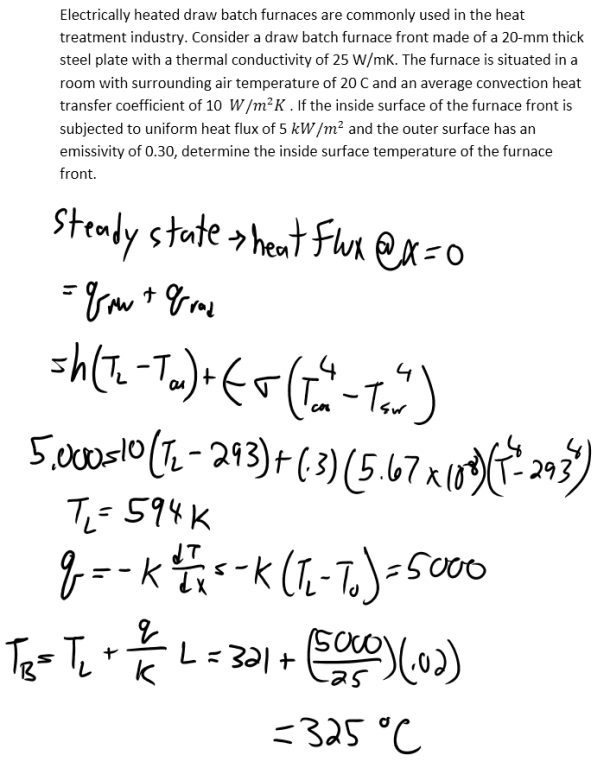heat and mass transfer problems engineering equations heat and mass transfer school homework engineering heat and mass transfer formulas heat and mass transfer solutions to heat and mass transfer problems full solution engineering problem solution heat and mass transfer math problems engineering equations heat and mass transfer school homework engineering solutions to heat and mass transfer formulas heat problems mass problem solutions to transfer problems full solution heat and mass transfer
heat and mass transfer problems engineering equations heat and mass transfer school homework engineering heat and mass transfer formulas heat and mass transfer solutions to heat and mass transfer problems full solution engineering problem solution heat and mass transfer math problems engineering equations heat and mass transfer school homework engineering solutions to heat and mass transfer formulas heat problems mass problem solutions to transfer problems full solution heat and mass transfer
Highalphabet Home Page heat and mass transfer problem solutions Heat and Mass Transfer Page
Electrically heated draw batch furnaces are commonly used in the heat treatment industry. Consider a draw batch furnace front made of a 20-mm thick steel plate with a thermal conductivity of 25 W/mK. The furnace is situated in a room with surrounding air temperature of 20 C and an average convection heat transfer coefficient of 10 W/m^2 K . If the inside surface of the furnace front is subjected to uniform heat flux of 5 kW/m^2 and the outer surface has an emissivity of 0.30, determine the inside surface temperature of the furnace front.Electrically heated draw batch furnaces are commonly used in the heat treatment industry. Consider a draw batch furnace front made of a 20-mm thick steel plate with a thermal conductivity of 25 W/mK. The furnace is situated in a room with surrounding air temperature of 20 C and an average convection heat transfer coefficient of 10 W/m^2 K . If the inside surface of the furnace front is subjected to uniform heat flux of 5 kW/m^2 and the outer surface has an emissivity of 0.30, determine the inside surface temperature of the furnace front.